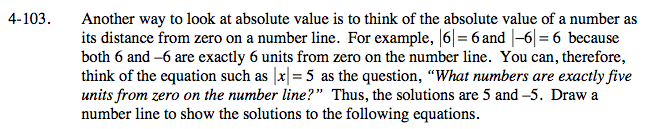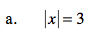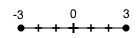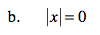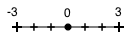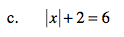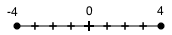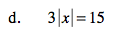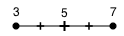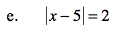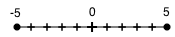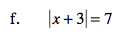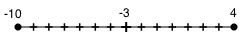### Home > A2C > Chapter Ch4 > Lesson 4.2.3 > Problem4-103

4-103.
1. Another way to look at absolute value is to think of the absolute value of a number as its distance from zero on a number line. For example, |6| = 6 and |−6| = 6 because both 6 and “6 are exactly 6 units from zero on the number line. You can, therefore, think of the equation such as |x| = 5 as the question, “What numbers are exactly five units from zero on the number line?” Thus, the solutions are 5 and −5. Draw a number line to show the solutions to the following equations. Homework Help ✎

1. |x| =3

2. |x| = 0

3. |x| + 2 = 6

4. 3|x| = 15

5. |x − 5| = 2

6. |x + 3| = 7